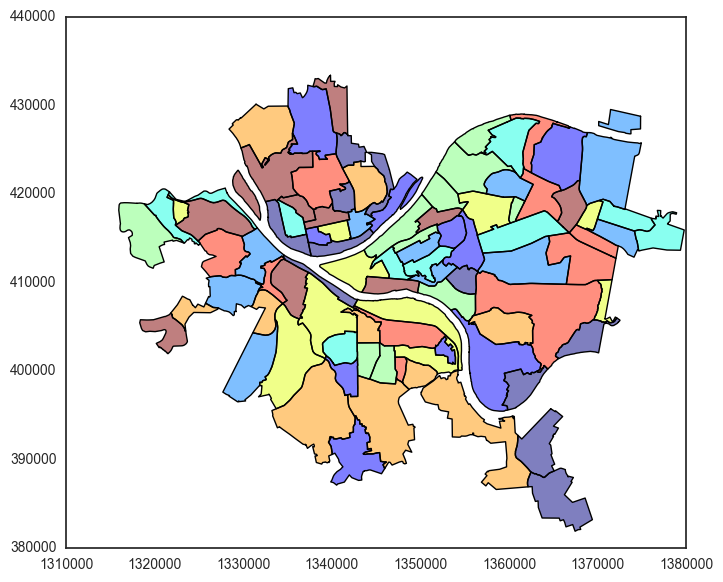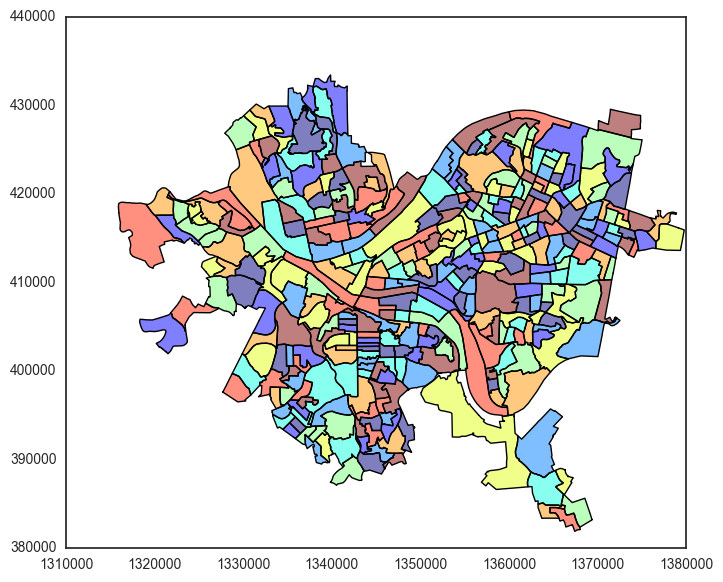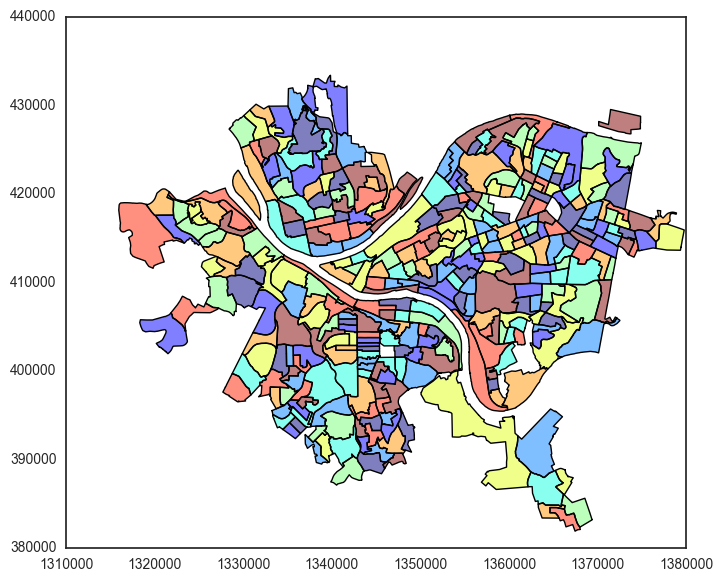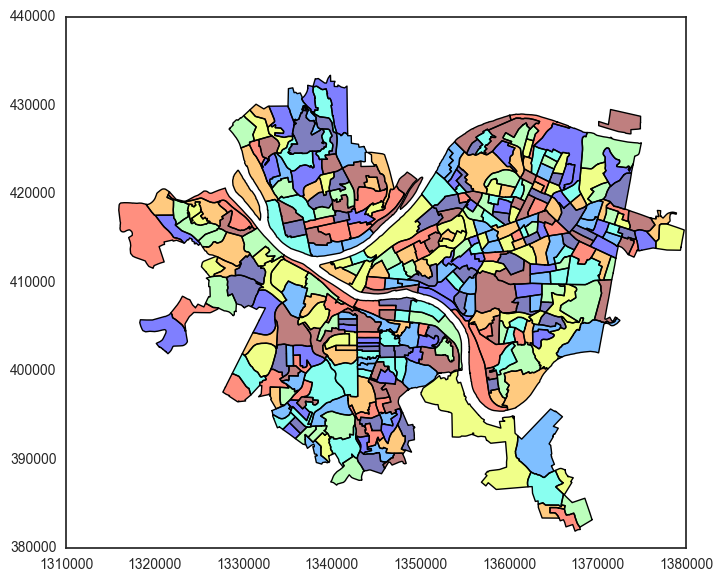## Introduction¶

This tutorial will introduce you to some basic methods for processing geospatial data, particularly focusing on Geographic Information Systems (GIS) data. Geospatial data forms a key aspect of data science. In many settings we want to visualize or analyze data with a spatial component: where (geographically) are certain features located, how do certain properties correlate with location, and can we identify spatial patterns in data? Sometimes this also involves substantial analysis or machine learning, but sometimes just visuallizing data spatially tells us a great deal about some question we are interested in.

A classical example is the following map, created by John Snow in 1854:(click for full-size version). This is map of London in the 19th century, as it was experiencing a Cholera outbreak. John Snow took a map of the streets of London, and plotted the number of Cholera death spatially. What emerged is that the deaths were clearly centered around a pump on Broad Street. This visual evidence was enough to persuade the government council to remove the handle to the pump, and provided substantial evidence that Cholera was being spread by contaminated water (the well was later found to have been contaminated by sewage).

### Tutorial content¶

In this tutorial, we will show how to do some basic spatial analysis in Python, specifically using GeoPandas, Shapeley, and GeoPy.

We'll be using data collected from the Pittsburgh GIS data repository from the Pittsburgh city planning department: http://pittsburghpa.gov/dcp/gis/gis-data-new. While there aren't always public repositories for GIS data, a surprisingly large number of cities or counties maintain an up-to-date set of GIS data available on their web pages, and the analysis we do here could be repeated for many other cities as well.

We will cover the following topics in this tutorial:

## Installing the libraries¶

Before getting started, you'll need to install the various libraries that we will use. You can install GeoPandas, Shapely (automatically installed as a dependency of GeoPandas), and GeoPy using pip:

$pip install --upgrade geopandas geopy  On my machine, GeoPandas throws an exception in its spatial join command unless the Rtree library is also installed. There are instructions for installing Rtree on the web page above, but I was able to use the following command, which installed the relevant libraries: $ conda install -c ioos rtree



Note that this installs the rtree module from a private repository (called ioos), not an official conda package, so there is a chance it won't work for you, and you'll need to consult the documentation. After you run all the installs, make sure the following commands work for you:

In :
import geopandas as gpd
import shapely
import rtree
import numpy as np


Now that we've installed and loaded the libraries, let's load our first geographic data. We're going to load data provided in the "shapefile" format, which stores vector data that is geographically tagged (i.e., the points are expressed in terms of longitude/latitude or some other geographic coordinate system).

Download the Census_Data.zip file from the Pittsburgh city planning website: http://apps.pittsburghpa.gov/dcp/Census_Data.zip. Then unzip the Neighborhood.zip file to create a Neighborhood folder with a number of files inside, including a Neighborhood.shp file. This is the Shapefile we want, but in an odd quirk of the Shapefile format, you actually need all the files in this directory in order to properly load the file (GIS systems were developed before people knew how to properly create database files, apparently). So you'll want to copy the entire Neighborhood directory into the same folder as this notebook, and you can then load the data using the following command.

In :
df_neigh = gpd.read_file("Neighborhood/Neighborhood.shp");

Out:
ACRES ALAND10 AREA AWATER10 BLKGRPCE10 COUNTYFP10 DPWDIV FID_BlockG FID_Neighb FUNCSTAT10 ... SQMILES STATEFP10 Shape_Ar_1 Shape_Area Shape_Le_1 Shape_Le_2 Shape_Leng TRACTCE10 UNIQUE_ID geometry
0 180.883 268195.0 7843108.0 0.0 1 003 3 0 62 S ... 0.281 42 7.843108e+06 7.842997e+06 11526.863222 11525.904546 7988.379391 040500 113 POLYGON ((1355377.123158768 411531.0846695155,...
1 320.679 75472.0 13904629.0 0.0 1 003 3 1 40 S ... 0.499 42 1.390463e+07 1.390469e+07 20941.386025 20945.562570 3813.620989 040400 25 POLYGON ((1355110.970147774 417655.421174109, ...
2 138.372 282679.0 5999801.5 0.0 2 003 3 2 61 S ... 0.215 42 5.999801e+06 5.998649e+06 18271.426385 18280.484515 8260.915502 040200 21 POLYGON ((1352807.998047695 411926.938864693, ...
3 166.101 284548.0 7202139.0 0.0 2 003 3 3 56 S ... 0.258 42 7.202139e+06 7.203631e+06 15696.759230 15694.771443 8670.428122 030500 19 POLYGON ((1347150.050702184 413333.3376266807,...
4 390.864 1593129.0 16947852.0 329216.0 1 003 1 5 27 S ... 0.608 42 1.694785e+07 1.694875e+07 23903.077852 24019.532672 24019.532865 020300 23 POLYGON ((1349414.006476104 416876.8987810165,...

5 rows × 32 columns

The df_neigh object is of type GeoDataFrame, a subclass of a Pandas DataFrame, so you can use all the same calls you would use with a Pandas DataFrame. If you select a column from the object (other than the special "geometry" data type referenced below), it will simply be a normal Pandas Series. For example, if we wanted to get the total square miles over all neighborhoods, we could do so with the command:

In :
df_neigh.loc[:,"SQMILES"].sum()

Out:
53.126000000000005

The one special element of a GeoDataFrame is that it has one additional column with the name "geometry". This one column is a GeoSeries type, and contains a series of Shapely geometry objects. We won't worry about using Shapely itself too much except to generate basic geometry types, for the case of the df_neigh object, each entroy of "geometry" contains a Shapely Polygon type, which describes the geometry of the neighborhood as a list of points.

In :
g = df_neigh.loc[0,"geometry"]
print(str(g)[:100] + "...")

POLYGON ((1355377.123158768 411531.0846695155, 1355382.612321019 411522.5715631843, 1355390.25764694...


These points describe the outline of this speicfic neighborhood in Pittsburgh (the name is in the "HOOD" column), and this geometric information lets us plot the DataFrame visually. Specifically, we can use the .plot() command to visualize the geometry of the entire DataFrame. In this case, we can plot the data to view the neighborhoods of Pittsburgh visually:

In :
import matplotlib.pyplot as plt
import seaborn
seaborn.set_style("white")
%matplotlib inline

/Users/zkolter/anaconda/lib/python2.7/site-packages/matplotlib/__init__.py:878: UserWarning: axes.color_cycle is deprecated and replaced with axes.prop_cycle; please use the latter.
warnings.warn(self.msg_depr % (key, alt_key))

In :
df_neigh.plot(figsize=(8,8), cmap="jet");## Geometric operations¶

While the ability to simply plot the data is nice, is nice, one of the powerful aspects of these geometric tools is the ability to perform spatial operations on the data. For example, lets consider an alternative breakdown of the Pittsburgh area by census block groups. These breakdowns are contained in Block_Group_2010.zip file folder as above. Let's load the shapefile as before, and then plot it to visualize these breakdowns.

In :
df_block_group = gpd.read_file("Block_Group_2010/Block_Group_2010.shp");
df_block_group.plot(figsize=(8,8), cmap="jet");This looks ok, but unlike the neighborhood shapes, these block groups cover the river areas in Pittsburgh, so they don't look quite as nice as what we had previously. To address this, we're going to compute the intersection between each polygon in the df_block_group data frame and the union of all the neighborhoods (a an object we can get using the .unary_union property of a GeoDataFrame). We can do this using the .intersection() command:

In :
df_block_group["geometry"] = df_block_group.intersection(df_neigh.unary_union)
df_block_group.plot(figsize=(8,8), cmap="jet");This looks good, but as a minor point, it looks like there are a few holes in this map. What's happening here is that some of the intersections are resulting in GeometryCollection types instead of Polygon or MultiPolygon types (you can check this by looking at the type of each geometry). This is likely a minor bug in the intersection code, and you can fix it by simply only keeping the polygons from the intersections, as follows.

In :
is_collection = lambda x : isinstance(x, shapely.geometry.collection.GeometryCollection)
to_multipolygon = lambda x : shapely.geometry.MultiPolygon([p for p in x if isinstance(p, shapely.geometry.Polygon)])

idx = df_block_group["geometry"].apply(is_collection)
df_block_group.loc[idx,"geometry"] = df_block_group.loc[idx,"geometry"].apply(to_multipolygon)
df_block_group.plot(figsize=(8,8), cmap="jet");Much nicer. The .intersection() is just one possible geometric operation. We can also create unions between separate geometries (we already effectively did this with the .unary_union property), differences, symmetric differences, etc.

## Spatial joins¶

Similar to geometric operations, but also integrating the more relational components of GIS data is the notation of a "spatial join". Just like traditional join operators merge tables based upon the value of certain columns in these two tables, a spatial join merges together two geospatial tables based upon properties of their geometry entries. In a spatial join we merge together two rows that satisfy some geometric property between the rows. The most obvious case is that we can, for instance, merge together rows where one geometry intersects with the other, or where one geometry is contained within the other.

This idea is best demonstrated through an example. Let's suppose that we would like to build a map of the fair market value of residential homes within Pittsburgh, organized by census block group. To get housing data, we'll download the Land_Management.zip file (http://apps.pittsburghpa.gov/dcp/Land_Management.zip) from the Pittsburgh GIS data, and specifically look at the Parcels shapefiles.

In :
# Load the Parecels shapefile (note: this is a big file, and takes about a minute)